Computation of the n'th digit of pi in any base in O(n^2)

Fabrice Bellard

Simon Plouffe explained in  a new algorithm to compute the n'th digit ofand some other mathematical constants in any base with very little memory. Its running time is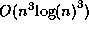. We present here an improvement of this algorithm whose running time is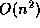while its memory requirements stay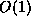, which makes it practical to compute the millionth digit offor example.

1 First result

We want to compute the n'th digit in base B of s, where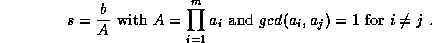Let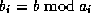,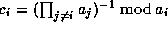and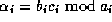. We have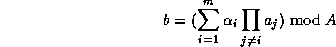with the Chinese remainder theorem. Hence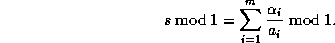The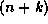'th digits of s, where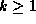, are the digits in base B of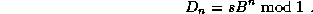With the last formula we have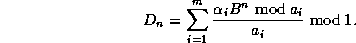As shown in , this equality is interesting because each term can be computed separatly, with a total memory ofif we already know b and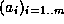.

2 Second result

We want now to compute the n'th digit in base B of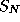, where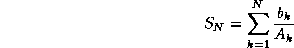with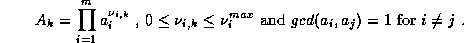With the first result we have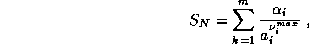where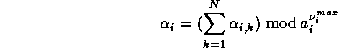with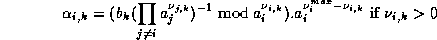and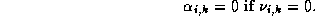The key observation is that we can use for all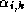the same modulo, hence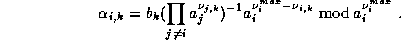This can be rewritten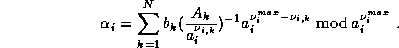To have the digits after the n'th one, in base B, we compute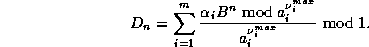The running time is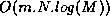and the memory requirements stayif we suppose that:

•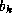,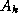,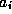,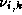and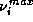are given.

•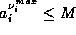for every i. We suppose that M fits in a computer word so that each operation takes a time ofexcept the modulo inversion which takes a time of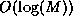.

•is small so an exponentiation by it takes a time of.

3 Application

Given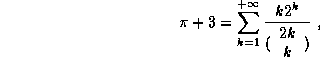we can use the result of section 2 because if p is a prime number, we notice that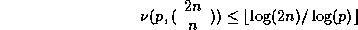where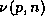is the multiplicity of p in n. It comes from the relation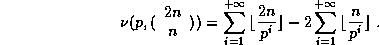Hence, if we want the n'th digit ofin base B, we may use the following algorithm:

•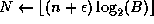whereis a small integer to ensure we have the precision needed ;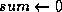.

• For each prime number a with 2 < a < 2N, do:

•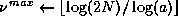;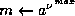.

•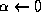;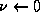;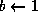;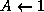.

• for k in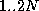do:

•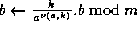;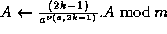;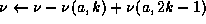.

• if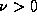do: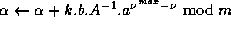.

•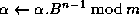;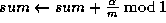.

• If we suppose that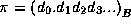, then, if we neglect rounding errors,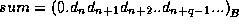. The number q of correct digits depends on.

The running time isbecause there are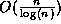prime numbers between 2 and 2n . The memory requirements are, as expected, in.

4 Conclusion

We have presented an algorithm to compute the n'th digit in any base B ofwhose running time is. It has the same running time as other classical methods for computing(e.g. arctangent formulas), but it uses little memory, it is very simple and does not need high precision computations. It is still slower than the BBP algorithm , but it works in any base. As described in , the same algorithm may be used to compute other numbers such as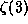,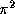,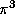,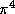and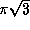.

References

1
Simon Plouffe, On the computation of the n'th decimal digit of various transcendental numbers, November 1996.

2
David H. Bailey, Peter B. Borwein and Simon Plouffe, On the Rapid Computation of Various Polylogarithmic Constants, April 1997 in Mathematics of Computation.

Sun Jan 12 22:34:36 MET 1997
Fabrice Bellard ( http://bellard.org/ )